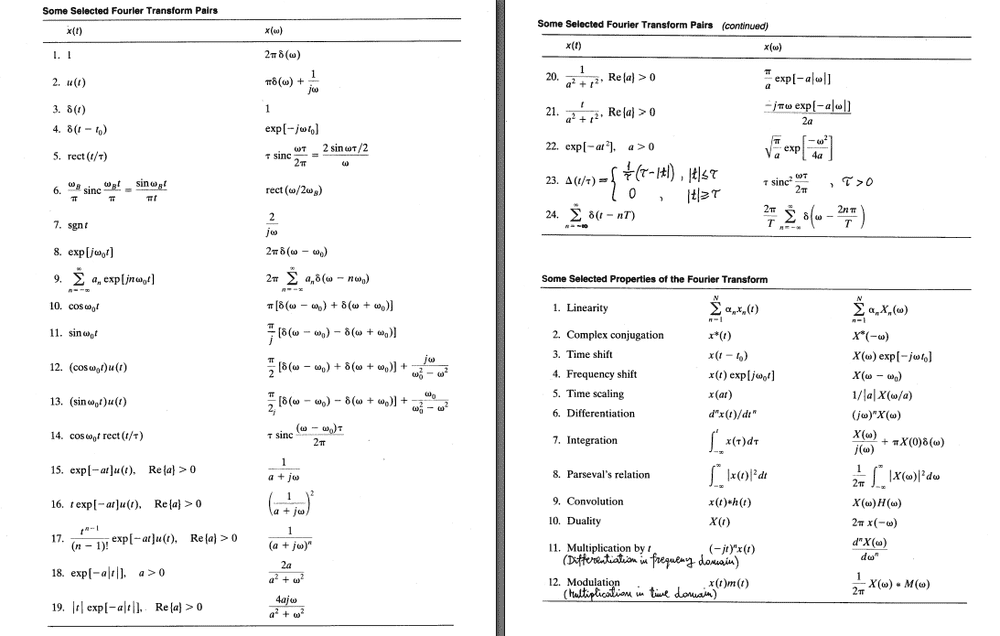# Calculation of Fourier Transform Derivative d/dw (F{x(t)})=d/dw(X(w))

Alexei_Nomazov
TL;DR Summary
Calculation of Fourier Transform Derivative d/dw (F{x(t)})=d/dw(X(w))
Calculation of Fourier Transform Derivative d/dw (F{x(t)})=d/dw(X(w))
Hello to my Math Fellows,

Problem:
I am looking for a way to calculate w-derivative of Fourier transform,d/dw (F{x(t)}), in terms of regular Fourier transform,X(w)=F{x(t)}.

Definition Based Solution (not good enough):
fromI can find that w-derivative of Fourier transform for x(t) is Fourier transform of t*x(t) multiplied by -j:
d/dw (F{x(t)})=d/dw(X(w))=-j*F{t*x(t)}

Question:
But, taking into account the differentiation and duality properties of Fourier transform:is it possible to express the derivative, d/dw (F{x(t)}), in frequency domain using terms of X(w) ?

Many Thanks,
Desperate Engineer.

Gold Member
First I want to clarify your question, since I find your notation to be complicated. If ##X(\omega)## is the Fourier transform of ##x(t)##, are you asking if there is a way to write ##\frac{d}{d\omega} X(\omega)## in terms of ##X(\omega)##?

If you are looking for an actual formula, then the answer is no. Consider a couple of examples. If ##X(\omega)=1##, then ##\frac{d}{d\omega} X(\omega) = 0 = 0 \, X(\omega)##; on the other hand if ##X(\omega) = e^{j \omega \tau}## then ##\frac{d}{d\omega} X(\omega) = j\tau\,e^{j \omega \tau} = j\tau\, X(\omega)##. Fourier transforms are just functions - and when you learned calculus you had to learn a bunch of different examples of derivatives (polynomials, sinusoids, exponentials, etc) - so it shouldn't be a surprise that there isn't a simple expression like you seem to be looking for.

On the other hand, if you just want a symbolic expression that allows you to write it a slightly different way, then you can just write ##\frac{d}{d\omega} X(\omega) = \delta^\prime(\omega) \ast X(\omega)##, where ##\delta^\prime(\omega)## is the derivative of the delta function, and ##\ast## represents convolution. But this is just using the definition of the delta function and convolution, and doesn't actually help you compute anything.

Good luck,

jason

•Alexei_Nomazov
Gold Member
Well, when you learned calculus did you learn one single formula that gives you the derivative of any arbitrary differentiable function in terms of the original function? Yes, but it is just the definition of derivative, which probably isn't what you are looking for.

Perhaps if you tell us what you are actually trying to do we could help.

jason

Alexei_Nomazov
Thank you Jason.
I think i was misleading myself a little bit reducing my problem to strict technical one.